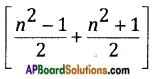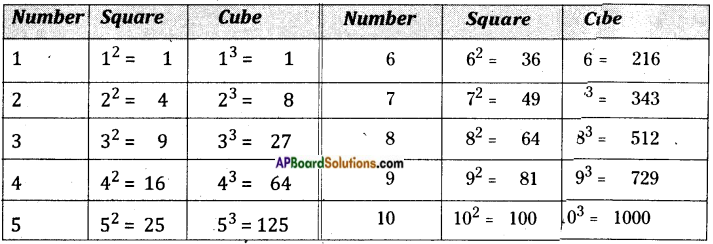Students can go through AP Board 8th Class Maths Notes Chapter 6 Square Roots and Cube Roots to understand and remember the concepts easily.

## AP State Board Syllabus 8th Class Maths Notes Chapter 6 Square Roots and Cube Roots

→ The product of two same numbers is called its square.
Ex: 1) x × x = x2
2) 6 × 6 = 62 = 36

→ The digits in the units place of a square number are 0, 1, 4, 5, 6, 9.

→ If the digits 2,3, 7 or 8 are in the units place of an umber then it is not a perfect square.→ If there are ‘n’ digits in a number then the no.of digits in its square = 2n or (2n -1).

→ Sum of ‘n ‘ consecutive odd numbers = n2

→ The square of any odd number say ‘n’ can be expressed as the sum of two consecutive numbers as→ If a, b, c are any three positive integers and a2 + b2 = c2 then a, b, c are called Pythagorean triplets. Ex: (3, 4, 5) (5, 12, 13).

→ If a square number is expressed, as the product of two equal factors, then one of the factors is called the square root of that square number. Thus, the square root of 169 is 13. It can be expressed as √169 = 13 (symbol used for square root is √). Thus it is the inverse operation of squaring.

→ If the same number is multiplied itself by 3 times then it is called a cube of a number. Ex: cube of x = x × x × x = x3→ If a cube number is expressed, as the product of 3 equal factors, then one of he factors is called the cube root of that number.
Symbol for cube root is $$\sqrt{ }$$
Ex: $$\sqrt{64}=\left(4^{3}\right)^{1 / 3}$$ = 4